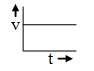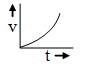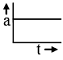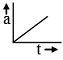Ex﻿

# 1. Motion

#### Various Graphs Related To Motion

Displacement - Time Graph :
The straight line inclined to time axis in s-t graph represents constant velocity.In s-t graph the straight line inclined to time axis at angle greater than 90º shows negative velocityBody with accelerated motionBody with decelerated motionVelocity - Time Graph :
For the body having constant velocity or zero acceleration.The body is moving with constant retardation and its initial velocity is not zero.The body is accelerated and the initial velocity is zero.The body is deceleratedAcceleration-time graph :
Acceleration is constantAcceleration is increasing and is +veAcceleration is decreasing and is –veIf you want to give information about online courses to other students, then share it with more and more on Facebook, Twitter, Google Plus. The more the shares will be, the more students will benefit. The share buttons are given below for your convenience.
×

#### NTSE Physics (Class X)

• Electricity
• Magnetic Effect of Curent
• Light Reflection
• Light Refraction
• Human Eye & Colourful World
• Source of Energy
• Motion

#### NTSE Physics (Class IX)

• Force & laws of motion
• Work and Energy
• Gravitation
• Sound

SHOW CHAPTERS